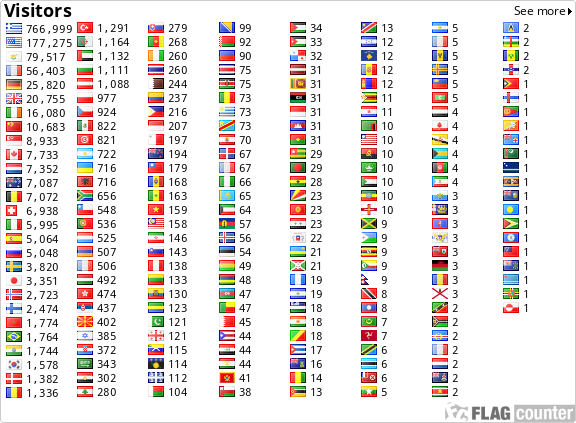﻿Posets, Groups and Hypergroups - N. Lygeros

Posets, Groups and Hypergroups

N. Lygeros

 Abstract – Via the transposition of the theorems of Birkhoff, Chaunier-Lygeros and Lygeros-Mizony on Groups to P-Hypergroups, we explicit some relations between posets and hypergroups. 1. Definitions is a hypergroup if : H*H→p(H) is an associative hyperoperation for which the reproduction axiom hH=Hh=H is valid for every h of H . A hypergroup (H,.) is called cyclic with finite period with respect an element h of H if there exists an integer v such that H=h1∪h2∪...∪hv. Let (G,.) be a group and P any non-empty subset of G. Then the P-hyperoperation P* is defined as follows: P*: G*G→p(G) : (x,y)→xP*y=xPy. If the reproduction axiom is valid then the hyperstructure is a P-hypergroup . 2. Theorems Theorem Birkhoff : If G is group then there exists a poset which automorphisms group is isomorphic to G. Theorem Birkhoff : If G is a finite group of cardinal a then there exists a poset which automorphisms group is isomorphic to G and with cardinal is equal to a2+a. Theorem Chaunier-Lygeros : Let a a prime number and n the minimal number of vertices of posets which have a automorphisms group of cardinality a. Then: (i)         n=a if a=2; (ii)        n=3a if a=3,5 or 7; (iii)       n=2a if a>7. Posets which realize the minimum and have a minimal number of relations are respectively: (i)       ({x0,x1}) with x0 and x1 incomparable; (ii)       ({x0,…,xa-1,y0,…ya-1,z0,…,za-1}) with xi< yi< zi and xi< zj if j-i≡1 (mod a); (iii)      ({x0,…,xa-1,y0,…ya-1}) with xi< yj if j-i≡0,1 or 3 (mod a). Theorem Lygeros-Mizony : If G is a finite group of cardinal a, non direct product of two groups, generated by elements which are two by two of distinct order then there exists a poset which automorphisms group is isomorphic to G and with cardinal is equal to 3a. 3. New Theorems Theorem 3.1: A poset Po can be associated to every P-hypergroup. Proof: By the definition of a P-hypergroup we have: G←→< G,P* > P-hypergroup and with the theorem of Birkhoff  we can associate a poset Po to a group G via its automorphisms group Aut(Po). So we have: Po ←→ Aut(Po) ≈ G←→ < G,P* > Q.E.D. Theorem 3.2: A poset Po of cardinality a2+a can be associated to every P-hypergroup with G of cardinality a. Proof: Corollary of the second theorem of Birkhoff.Q.E.D. Theorem 3.3: Let a a prime number, P-hypergroup and G a group of cardinality a=2 or 3,5,7 or 11 and more then there is an associated poset Po of cardinality respectively a or 3a or 2a which has an automorphism group of order a. Proof: Corollary of the theorem of Chaunier-Lygeros. Q.E.D. Theorem 3.4: Let P-hypergroup and G a finite group of cardinal a, non direct product of two groups, generated by elements which are two by two of distinct order then there exists a poset which automorphisms group is isomorphic to G and with cardinal is equal to 3a. Proof: Corollary of the theorem of Lygeros-Mizony. Q.E.D. 4. Questions Question 1: Can we associate a general hypergroup to a poset? Question 2: Can we associate a poset to a general hypergroup? References  Birkhoff G., 1946. Sobre los grupos de automorphimos. Revista Union Math. Arg., 11. pp.155-157.  Chaunier C. and N. Lygeros 1994. Posets minimaux ayant un groupe d’automorphismes d’ordre premier. C.R.Acad.Sci. Paris, t.318, Série I, p. 695-698.  De Salvo M. and Freni D. 1981. Semi-ipergruppi ciclici. Atti Sem. Mat. Fis. Un. Modena, 30, pp.44-59.  Lygeros N. and Mizony M.1996. Construction de posets dont le groupe d’automorphismes est isomorphe à un groupe donné. C.R.Acad.Sci. Paris, t.322, Série I, pp. 203-206.  Marty F. 1934. Sur une généralisation de la notion de groupe. 8th Congress Math. Scandinaves, Stockholm, pp.45-49.  Vougiouklis Th. 1990. Isomorphisms on P-hypergroups and cyclicity. Ars Combinatoria 29A , pp.241-245.

TexteOpus